# CBSE Class 4 Mathematics Sample Paper Set V

Read and download PDF of CBSE Class 4 Mathematics Sample Paper Set V designed as per the latest curriculum and examination pattern for Class 4 issued by CBSE, NCERT and KVS. The latest Class 4 Mathematics Sample Papers have been provided with solutions so that the students can solve these practice papers and then compare their answers. This will help them to identify mistakes and improvement areas in Mathematics Standard 4 which they need to study more to get better marks in Grade 4 exams. After solving these guess papers also refer to solved Class 4 Mathematics Question Papers available on our website to build strong understanding of the subject

## Sample Paper for Class 4 Mathematics Pdf

Students can refer to the below Class 4 Mathematics Sample Paper designed to help students understand the pattern of questions that will be asked in Grade 4 exams. Please download CBSE Class 4 Mathematics Sample Paper Set V

### Mathematics Class 4 Sample Paper

CBSE Class 4 Mathematics Sample Paper Set V. Sample Papers are the very important for every student. The sample papers should be practiced to gain extra marks in examinations. The sample papers have been prepared based on summative assessment1 and summative assessment 2 pattern. The sample papers have been prepared based on pattern of last year examinations and as per latest changes in the syllabus. Students, teachers and parents can download all CBSE educational material and very well prepared worksheets from this website.  All CBSE educational material is developed by our panel of teachers, have also been submitted by CBSE teachers and students.

I. Fill in the blanks. ½ x10 = 5

a) The boundary of a circle is called its ____________________.

b) We make the table of 9 with the tables of 2 and _________.

c) One litre = _______ ml + 450 ml.

d) ____________ quarters make a half.

e) The biggest chord of a circle is the ___________________.

f) 5 jars in each row with four rows will have ____________ jars.

g) If the cost of 1 kg sugar is Rs 36, then the cost of 1/4 kg sugar is Rs_______

h) Observe the pattern : 230, 340, 450, ______

i) 8/8 = _______

j) _________ 20-Rupee notes make a 100-Rupee note.

II. Choose the correct answer and fill in the blanks: ½ x10 = 5

a) There are ________ halves in a whole. ( 4, 2, 1 )

b) 300 grams is less than a kilogram by __________ grams. ( 600, 100, 700 )

c) The radius of a circle is ____________ of its diameter. (1/2, 3/4,1/4)

d) If YES is written as ZFT, the secret code on NO is ________ ( MP, NP, OP )

e) 10 × _______ ml = one litre. ( 200, 50, 100 )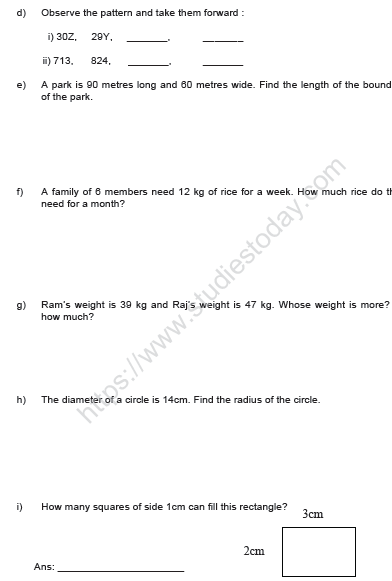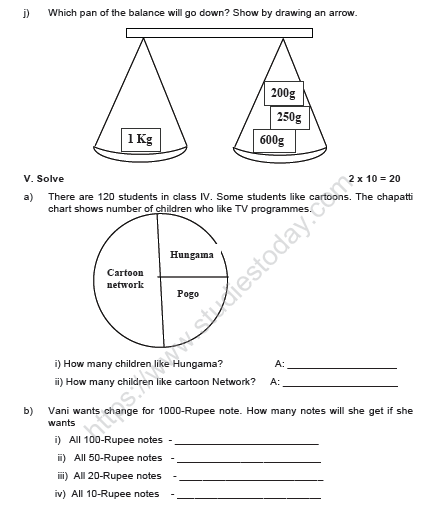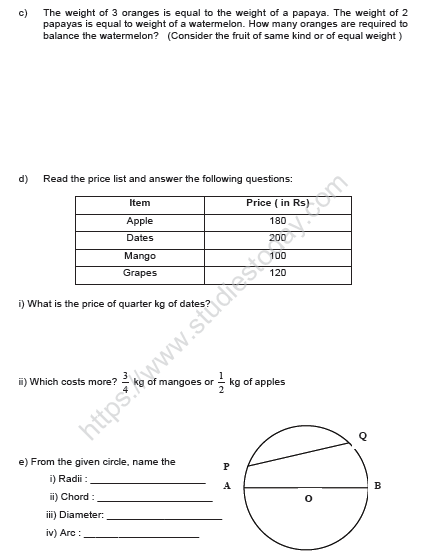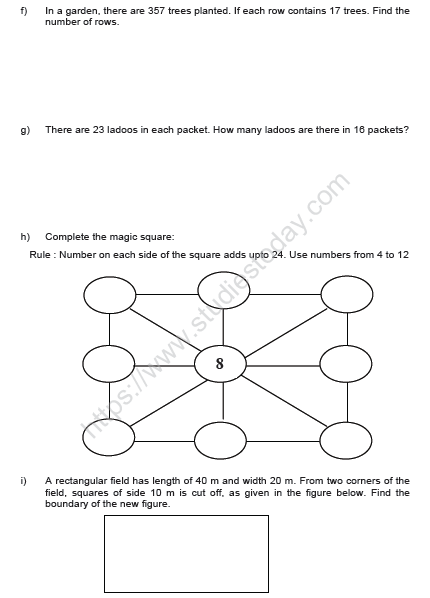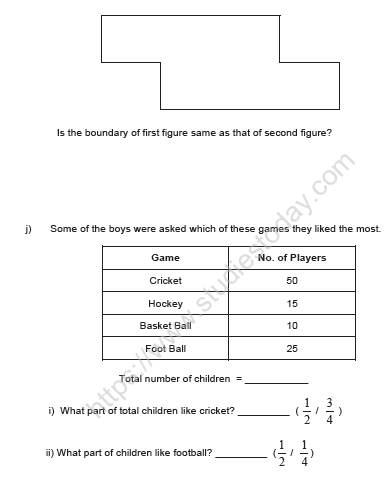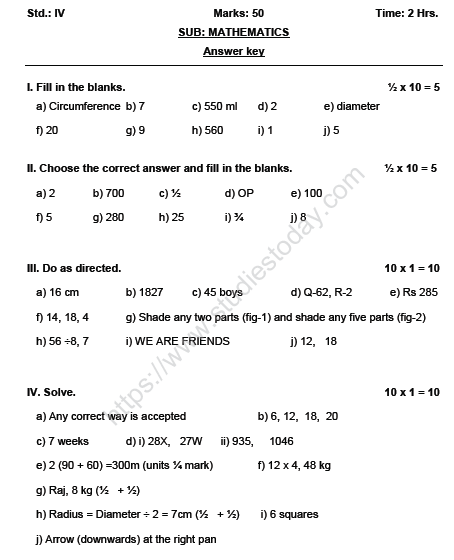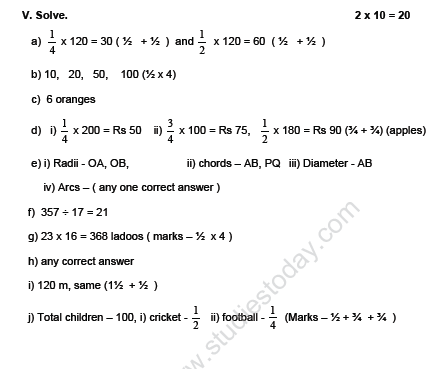Please click the below link to access CBSE Class 4 Mathematics Sample Paper Set V.